Electricity - Circuits and Graphs-2
10 MCQs questions on electricity
Email address *
Your Name *
Your answer
Class *
Your answer
Name of your school *
Your answer
Study the diagram and answer Q1.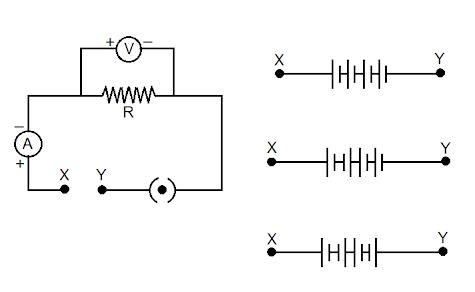1. To the terminals marked as X and Y in the given circuit, three students connect 4 cells of voltage 1.5 V each in three manners shown above: The readings of the voltmeter, for the three students, are likely to be (nearly) *
1 point
Read Q2 and study the table given below.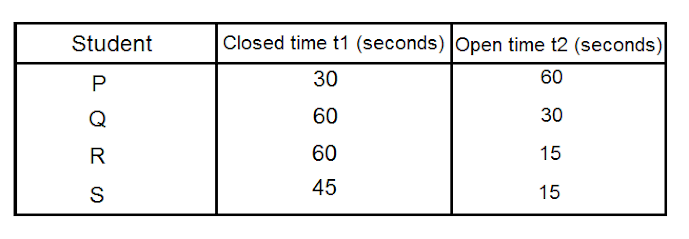2. In an experiment to study dependence of current I on the potential difference across a given resistor, students kept the plug key in the circuit closed for time t1 and then open for time t2. The times t1 and t2 for students P,Q ,R and S are given in the table above. The best choice of open and closed times is that of student: *
1 point
Study the diagram and answer Q3.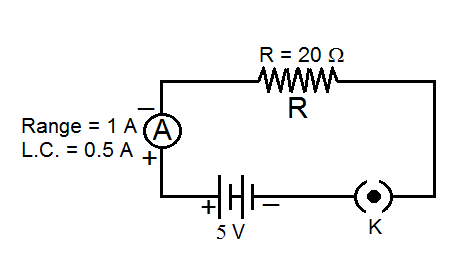3. On plugging the key K, the ammeter A reads zero. This is because *
1 point
Study the diagram and answer Q4.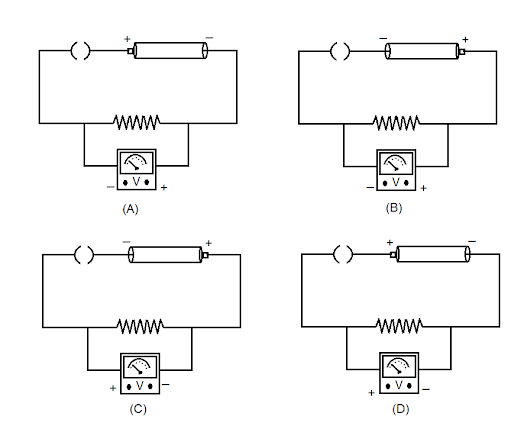4. The positive and negative terminals markings are shown in a circuit by the following figures. Which of the figures will give correct connections? *
1 point
Study the diagram and answer Q5.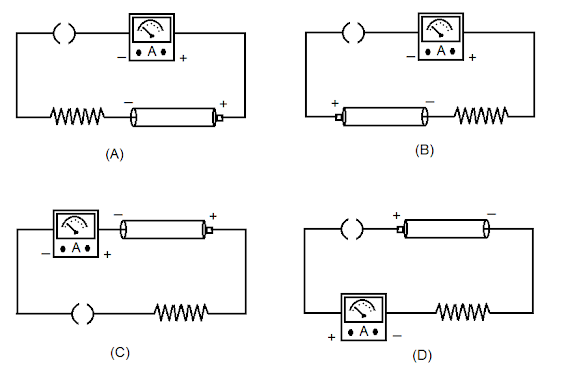5. The positive and negative terminal markings are shown in a circuit by the following figures. Which of the figures will give correct connections? *
1 point
6. The current varies linearly with the potential difference across a resistor. The slope of this variation will give *
1 point
Study the diagram and answer Q7.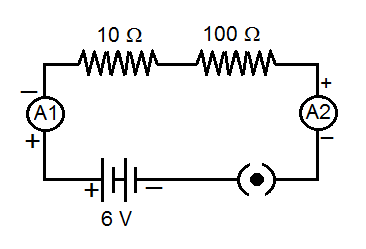7. In the above circuit, two ammeters are connected through two resistors in series whose pointer shows deflection. Then the reading of the ammeter A1 and A2 will be such that *
1 point
Study the diagram and answer Q8.8. In the above circuit, two voltmeters are connected through the resistors, whose pointer shows deflection. Then the reading of the voltmeters V1 and V2 will be such that
1 point
Study the diagram and answer Q9.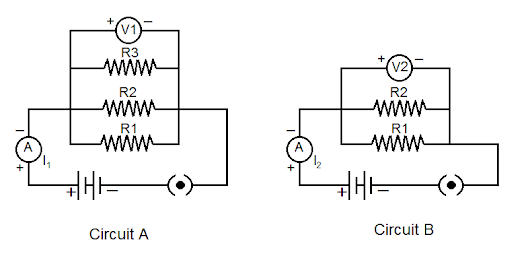9. . In Circuit A: Ammeter reads current I1 and the voltmeter reads voltage V1. In Circuit B: ammeter reads current I2 and voltmeter reads voltage V2. Both the circuits are connected to same voltage supply V. The relationship between the readings is *
1 point
Study the diagram and answer Q10.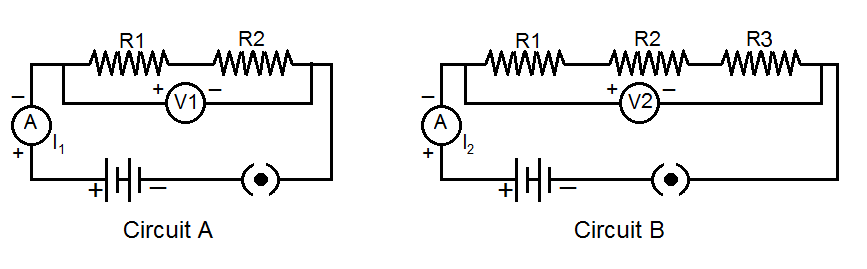10. If same voltage is supplied to both circuits A and B, then the relation between the ammeter and voltmeter readings in circuit A and B will be *
1 point
Never submit passwords through Google Forms.
This content is neither created nor endorsed by Google. Report Abuse - Terms of Service